# Elasticity of Input Substitution, Technological Progress and Income Distribution

#### A. The Elasticity of Input Substitution and the Shares of Factors of Production:

In this part we will examine how changes in factor prices affect the shares of factors and income distribution.

As factor prices change, the firm will substitute a cheaper input for a more expensive one. This profit-maximising behaviour will result in a change of the K/L ratio, and, hence, to a change in the relative shares of the factors.

The size of this effect depends on the responsiveness of the change of the K/L ratio to the factor price changes. A measure of this responsiveness is the elasticity of substitution. Recall that the elasticity of substitution is defined as the ratio of the percentage change in the K/L ratio to the percentage change of the MRTSL K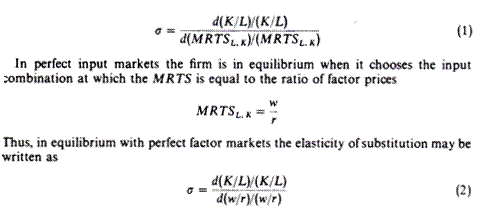The sign of the elasticity of substitution is always positive (unless σ = 0) because the numerator and denominator change in the same direction. When w/r increases, labour is relatively more expensive than capital and this will induce the firm to substitute capital for labour, so that the K/L ratio increases. Conversely a decrease in w/r will result in a decrease in the K/L ratio. The value of σ ranges from zero to infinity. If σ = 0 it is impossible to substitute one factor for another; K and L are used in fixed proportions (as in the input-output analysis) and the isoquants have the shape of right angles. If a =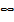the two factors are perfect substitutes: the isoquants become straight lines with a negative slope. If 0 < σ <factors can substitute each other to a certain extent the isoquants are convex to the origin. The Cobb-Douglas production function has a = 1. In general the larger the value of σ, the greater the substitutability between K and L.

We may classify σ in three categories:

σ < 1: inelastic substitutability

σ =1: unitary substitutability

σ > 1: elastic substitutability

There is an important relationship between the above values of a and the distribu­tive shares of factors. By definition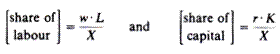Thus the relative factor shares are

Share of L / share of K = wL/rK

Share of L / share of K = (w/r)/(K/L)

From (3) we can easily find the effect of a change in the w/r ratio on the relative shares of the two factors.

Assume that σ < 1. This implies that a given percentage change in the w/r ratio results in a smaller percentage change in the K/L ratio, so that the relative-share expression increases. Thus, if σ < 1, an increase in the w/r ratio increases the distribu­tive share of labour. For example assume that σ = 0.5. Then a 10 per cent increase in w/r results in a 5 per cent increase in the K/L ratio.

The new relative shares are:If σ > 1 a change in w/r leads to a smaller percentage change in K/L, so that the relative share of labour decreases. For example assume that σ = 2. A 20 per cent increase in w/r leads to a 40 per cent increase in K/L The new share ratio is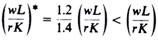Clearly if σ > 1 the relative share of labour decreases following an increase in the w/r ratio.

With a similar reasoning it can be shown that if a = 1 the relative shares of K and L remain unchanged.

In summary, an increase in the w/r ratio will cause labour’s share, relative to capital’s share, to

(a) Increase, if a < 1

(b) Decrease, if a > 1

(c) Remain the same, if a = 1

A decrease in the w/r share will have the opposite effects of the share of labour relative to capital’s share. It should be noted that there is a two-way causation between w/r and K/L Changes in the capital/labour ratio result in changes in the relative factor prices, and hence in changes in the shares of the factors to total output. The effects of changes of the K/L ratio on factor shares can be explored with the use of the elasticity of substitution. The analysis is analogous to the one we used above for changes in the w/r ratio.

From the above discussion it is clear that the concept of the elasticity of substitu­tion is very important in the neoclassical theory of income distribution. It is ex­tremely useful in examining the way in which changing input prices or input ratios affect income shares. Given that changes in w/r lead to changes in K/L and vice versa, it follows that predictions about factor shares following changes in one of these ratios are bound to be misleading if they do not take into account the possibility of the associated changes in the other ratio.

#### B. Technological Progress and Income Distribution:

We have assumed up to now a given production function. However, technological change takes place continuously, and this shifts the production function, leading to changes in the K/L ratio and the elasticity of substitution. Thus, it is important to consider the effects of technical progress on factor shares. We classified technological progress into three types, neutral, capital- deepening and labour-deepening. The relevant diagrams (figures 21.37, 21.38, 21.39) are reproduced for convenience.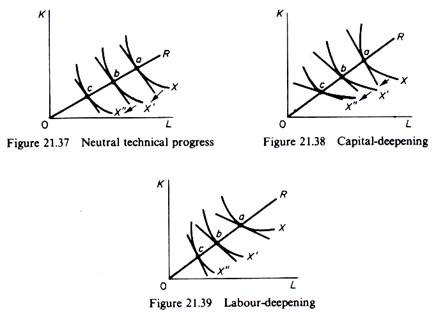Technological progress shifts the isoquants downwards, given that the same level of output can be produced with smaller quantities of factor inputs as technological progress occurs. In all three figures the isoquants X, X’ and X” represent the same level of output. OR is the ray whose slope shows a constant K/L ratio. Points a, b and c show the points of production at the given constant K/L ratio as technological progress takes place.

(a) Technological progress is neutral if at a constant K/L ratio the MRTSL,K remains unchanged. Since in equilibrium MRTSL, K = w/r it follows that when tech­nological progress is neutral both the K/L ratio and the w/r ratio are unchanged. Consequently the relative shares of factors remain unchanged.

(b) Technological progress is capital-deepening if at a constant K/L ratio the MRTSL, k declines. This implies that at equilibrium the w/r ratio declines, that is, r increases relative to w, while K/L remains constant.

Consequently the ratio of factor shares declines: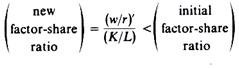This is tantamount to saying that the share of labour decreases and the share of capital increases.

(c) Technological progress is labour-deepening if at a constant K/L ratio the MRTSL, k increases. Then at equilibrium the w/r ratio increases as technological progress takes place. This implies that the share of labour will increase and the share of capital will decrease.

In summary, the relative share of labour:

(a) Increases if technological progress is labour-deepening,

(b) Remains unchanged if technological progress is neutral,

(c) Decreases if technological change is capital-deepening.

The opposite is true for the relative share of capital: this share decreases if technological change is labour- deepening, remains constant if technological change is neutral, increases if technolo­gical progress is capital-deepening.

The above analysis may be somewhat confusing, because it is based on Hicks’s definitions of the various forms of technical progress, which are expressed in terms of a constant K/L ratio. It is common knowledge that technological progress leads in general to a change of the K/L ratio. In fact K/L ratios have shown an upward trend over time in all industries, although the capital-deepening has proceeded at different rates in the various sectors.

This development has led Marxian economists to for­ecast an increase in capital’s share and, hence, a decrease in labour’s share. This prediction has not been realised. In most developed economies the factor shares have shown a remarkable stability over time. The increase in capital-deepening (K/L ratio) has resulted in increases of w/r, which, apparently, have not been taken into account in the above mentioned predictions. To avoid such misleading predictions it is preferable to analyse the effects of technological progress with the use of the elasticity of substitution.